Ex 10.3

Chapter 10 Class 11 Straight Lines
Serial order wise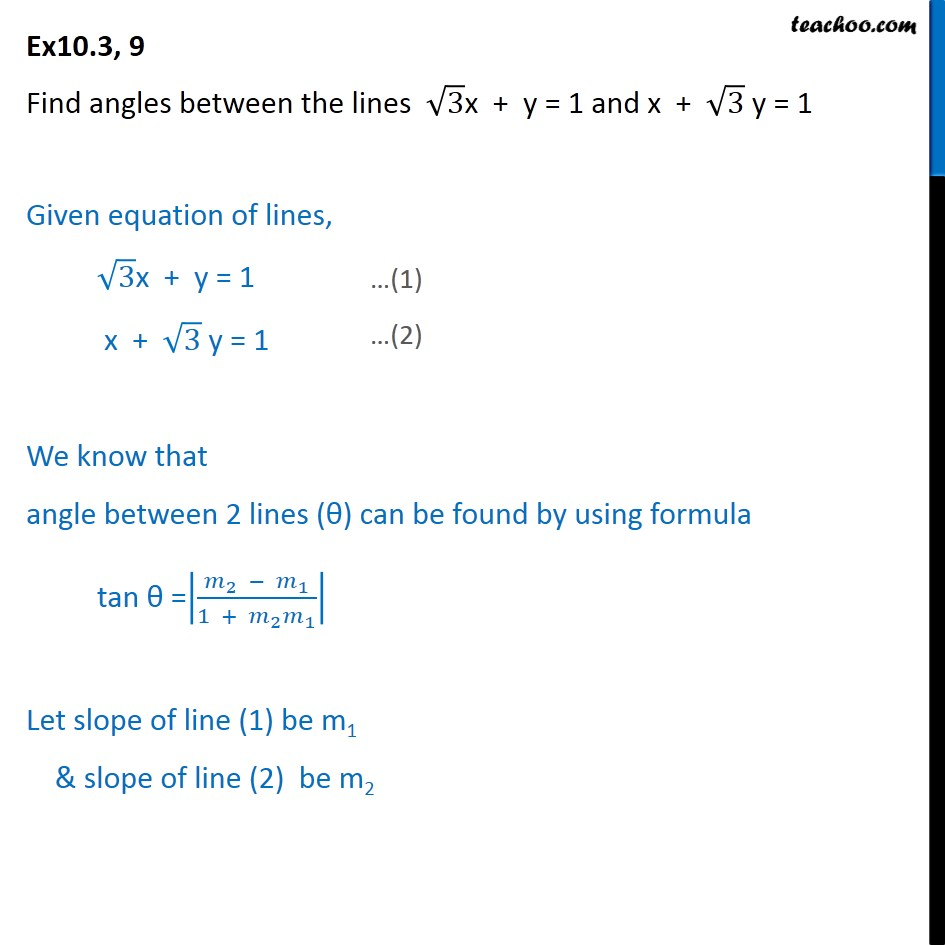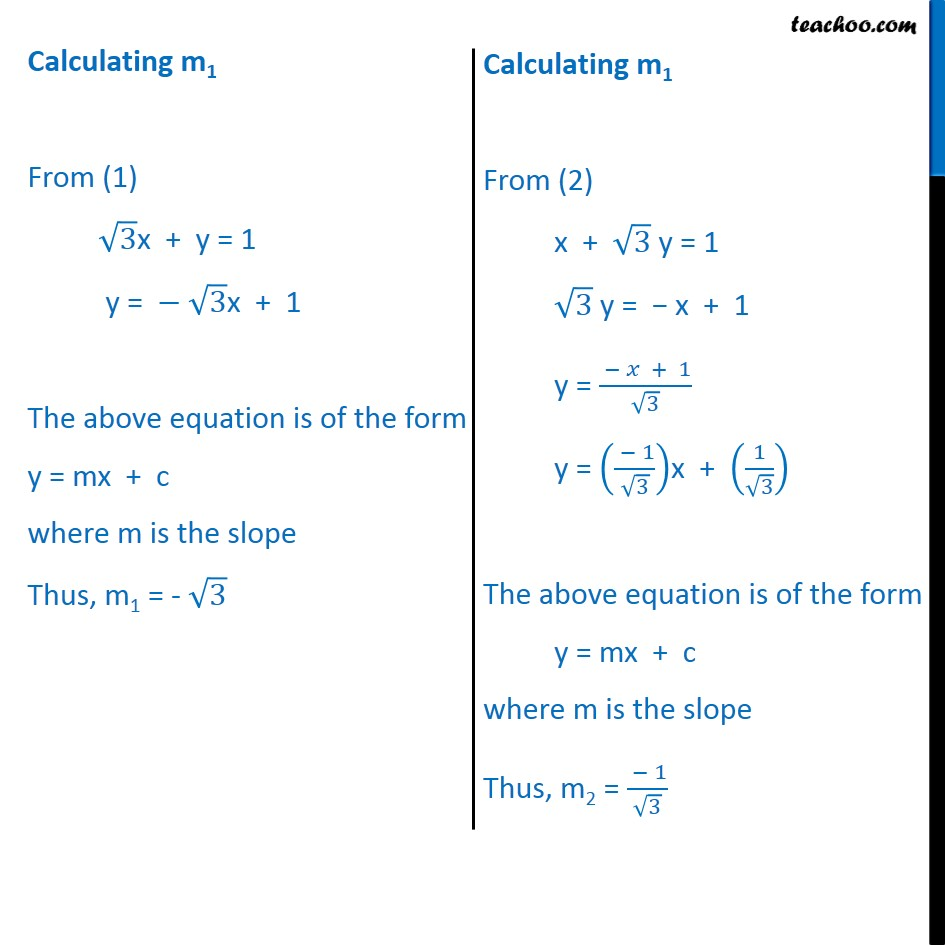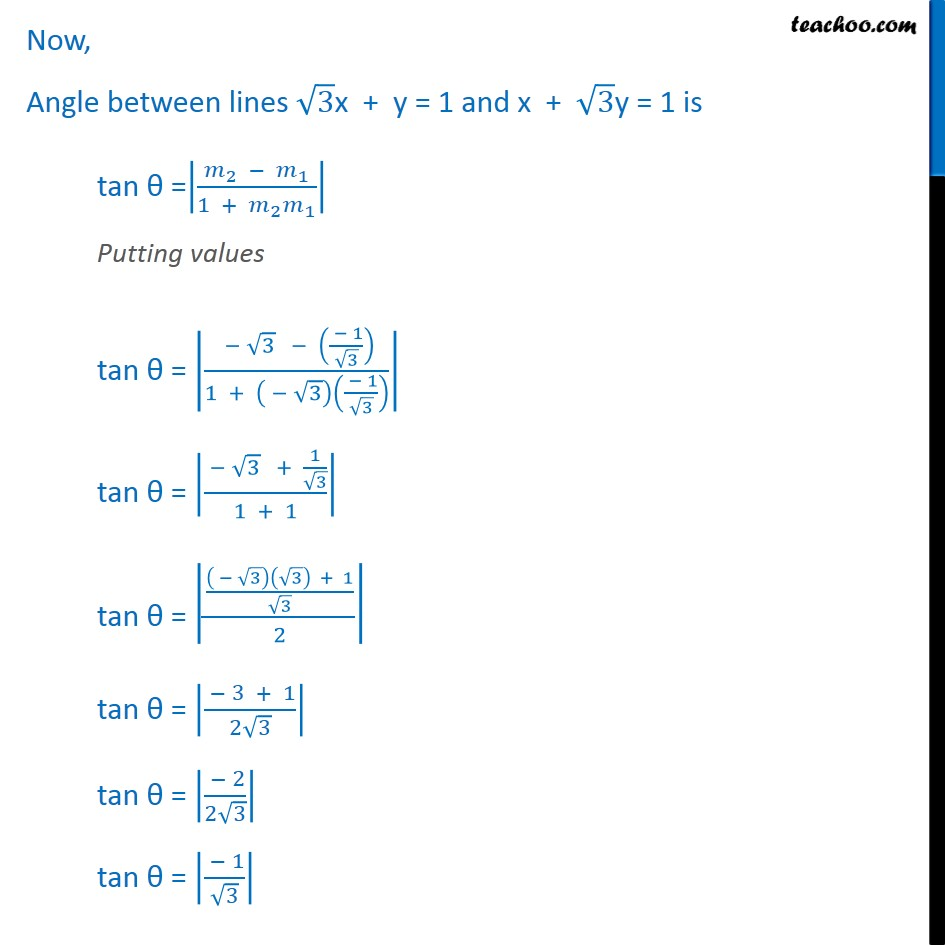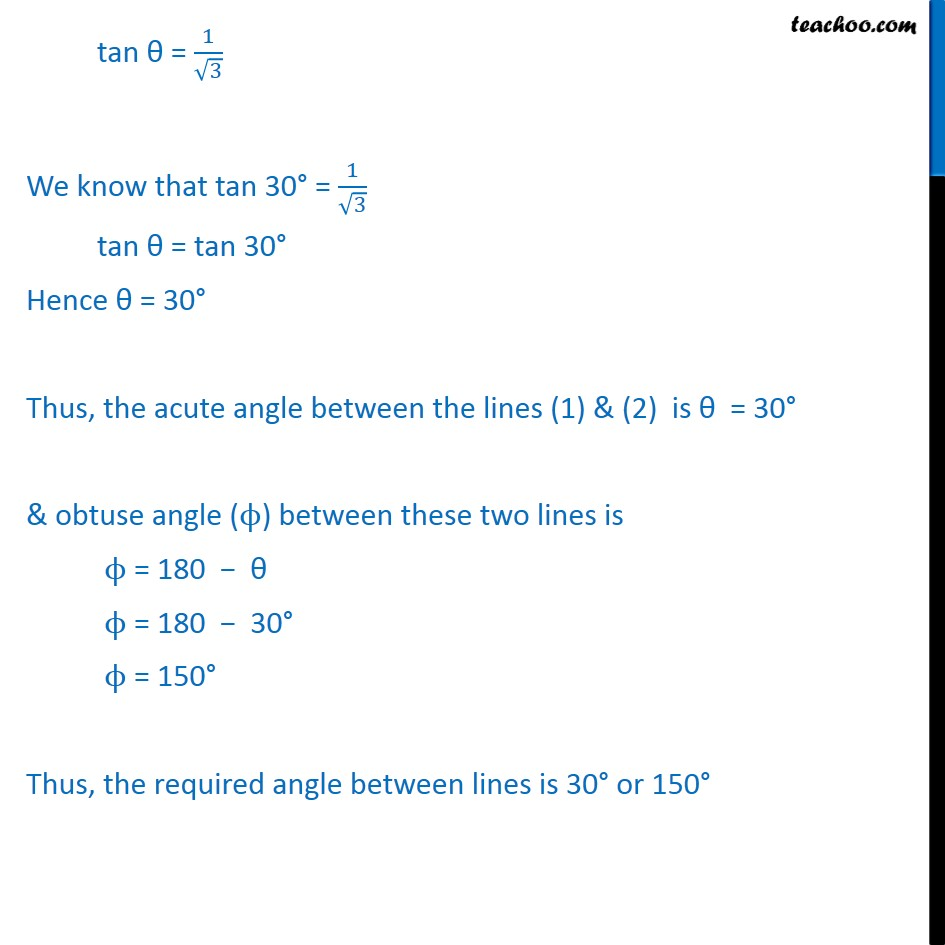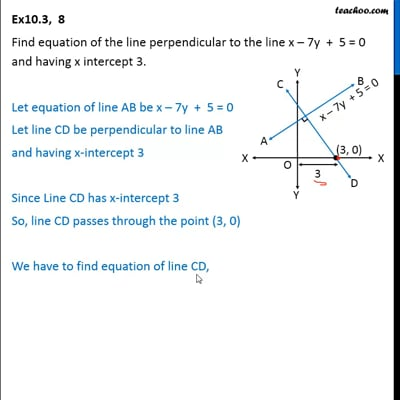This video is only available for Teachoo black users

Maths Crash Course - Live lectures + all videos + Real time Doubt solving!

### Transcript

Ex10.3, 9 Find angles between the lines 3x + y = 1 and x + 3 y = 1 Given equation of lines, 3x + y = 1 x + 3 y = 1 We know that angle between 2 lines ( ) can be found by using formula tan =|( _2 _1)/(1 + _2 _1 )| Let slope of line (1) be m1 & slope of line (2) be m2 Now, Angle between lines 3x + y = 1 and x + 3y = 1 is tan =|( _2 _1)/(1 + _2 _1 )| Putting values tan = |( 3 (( 1)/ 3))/(1 + ( 3)(( 1)/ 3) )| tan = |( 3 + 1/ 3)/(1 + 1)| tan = |((( 3)( 3) + 1)/ 3)/2| tan = |( 3 + 1)/(2 3)| tan = |( 2)/(2 3)| tan = |( 1)/ 3| tan = 1/ 3 We know that tan 30 = 1/ 3 tan = tan 30 Hence = 30 Thus, the acute angle between the lines (1) & (2) is = 30 & obtuse angle ( ) between these two lines is = 180 = 180 30 = 150 Thus, the required angle between lines is 30 or 150